# Decision trees¶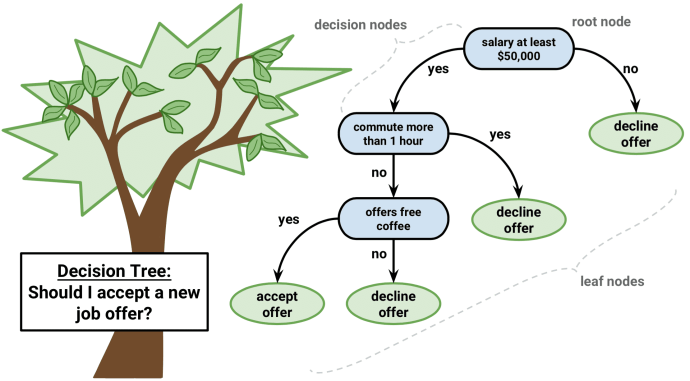Decision trees are a machine learning algorithm that predicts the value of a target variable based on decision rules learned from training data. The algorithm can be applied to both regression and classification problems by changing the objective function that governs how the tree learns the decision rules.

## How trees learn and apply decision rules¶

Decision trees learn and sequentially apply a set of rules that split data points into subsets and then make one prediction for each subset. The predictions are based on the outcome values for the subset of training samples that result from the application of a given sequence of rules.

The root is the starting point for all samples, nodes represent the application of the decision rules, and the data moves along the edges as it is split into smaller subsets until arriving at a leaf node where the model makes a prediction.

Each of these rules relies on one particular feature and uses a threshold to split the samples into two groups with values either below or above the threshold with respect to this feature.

During training, the algorithm scans the features and, for each feature, seeks to find a cutoff that splits the data to minimize the loss that results from predictions made using the subsets that would result from the split, weighted by the number of samples in each subset.

• Classification trees predict a probability estimated from the relative class frequencies or the value of the majority class directly.

• Regression models compute prediction from the mean of the outcome values for the available data points.

### Building Blocks of a Decision-Tree¶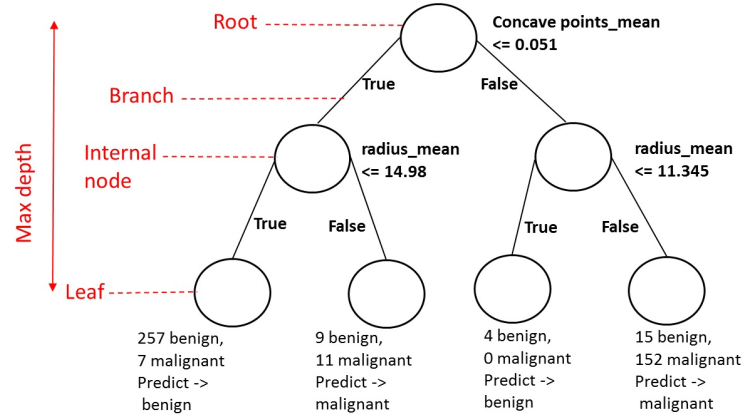• Node: question or prediction

Three kinds of nodes:

• Root: no parent node, question giving rise to two children nodes.
• Internal node: one parent node, question giving rise to two children nodes.
• Leaf: one parent node, no children nodes --> prediction.

#### Tree-based learning takes a top-down, greedy approach, known as recursive binary splitting.¶

• This process is recursive because it uses subsets of data resulting from prior splits.
• It is top-down because it begins at the root node of the tree, where all observations still belong to a single region and then successively creates two new branches of the tree by adding one more split to the predictor space.
• It is greedy because the algorithm picks the best rule in the form of a feature-threshold combination based on the immediate impact on the objective function rather than looking ahead and evaluating the loss several steps ahead.

The number of training samples gradually shrinks as recursive splits add new nodes to the tree. If rules split the samples evenly, resulting in a perfectly balanced tree with an $n$ equal number of children for every node, then there would be $2^n$ nodes at level $n$, each containing a corresponding fraction of the total number of observations. This is unlikely, so the number of samples along some branches may diminish rapidly, and trees tend to grow to different levels of depth along different paths.

To arrive at a prediction for a new observation, the model uses the rules that it inferred during training to decide which leaf node the data point should be assigned to. A smaller number of training samples in a given region of the feature space or leaf node, reduces the confidence in the prediction and may be overfitting.

• Splitting rules:
• mean (for regression)
• mode (for classification)

Recursive splitting continues until each leaf node contains only a single sample and the training error has been reduced to zero.

# How to use decision trees in practice¶

• To demonstrate regression trees we predict returns

• To demonstrate the classification case, we predict positive and negative asset price moves

## Imports¶

In :
%matplotlib inline

import warnings
import os
from pathlib import Path
import quandl
import numpy as np
import pandas as pd

import matplotlib.pyplot as plt
from matplotlib.ticker import FuncFormatter
from matplotlib import cm
import seaborn as sns
import graphviz
from IPython.display import Image
import pydotplus
from IPython.display import Image
from sklearn.tree import DecisionTreeClassifier, DecisionTreeRegressor, export_graphviz, _tree
from sklearn.linear_model import LinearRegression, Ridge, LogisticRegression
from sklearn.model_selection import train_test_split, cross_val_score, GridSearchCV, learning_curve, KFold
from sklearn.metrics import roc_auc_score, roc_curve, mean_squared_error, precision_recall_curve,  accuracy_score
import statsmodels.api as sm
from scipy.interpolate import interp1d, interp2d

In :
warnings.filterwarnings('ignore')
pd.set_option('display.max_columns', None)
plt.style.use('fivethirtyeight')


## Get Data¶

The data consists of daily stock prices for the $2010$-$2020$-$02$ period and various engineered features.

• The decision tree models here are not equipped to handle missing or categorical variables, so we applied dummy encoding to the latter after dropping any of the former.
• We also removed outliers for our response variable keeping data points in between the $5\%$ and $95\%$ quantiles respectively.
In :
with pd.HDFStore('data.h5') as store:
data = store['data']
data.info()

<class 'pandas.core.frame.DataFrame'>
MultiIndex: 22918 entries, (ABT, 2011-02-28 00:00:00) to (ZBRA, 2020-01-31 00:00:00)
Data columns (total 56 columns):
returns                   22918 non-null float64
t-1                       22918 non-null float64
t-2                       22918 non-null float64
t-3                       22918 non-null float64
t-4                       22918 non-null float64
t-5                       22918 non-null float64
t-6                       22918 non-null float64
t-7                       22918 non-null float64
t-8                       22918 non-null float64
t-9                       22918 non-null float64
t-10                      22918 non-null float64
t-11                      22918 non-null float64
t-12                      22918 non-null float64
year_2011                 22918 non-null uint8
year_2012                 22918 non-null uint8
year_2013                 22918 non-null uint8
year_2014                 22918 non-null uint8
year_2015                 22918 non-null uint8
year_2016                 22918 non-null uint8
year_2017                 22918 non-null uint8
year_2018                 22918 non-null uint8
year_2019                 22918 non-null uint8
year_2020                 22918 non-null uint8
month_1                   22918 non-null uint8
month_2                   22918 non-null uint8
month_3                   22918 non-null uint8
month_4                   22918 non-null uint8
month_5                   22918 non-null uint8
month_6                   22918 non-null uint8
month_7                   22918 non-null uint8
month_8                   22918 non-null uint8
month_9                   22918 non-null uint8
month_10                  22918 non-null uint8
month_11                  22918 non-null uint8
month_12                  22918 non-null uint8
size_1                    22918 non-null int8
size_2                    22918 non-null int8
size_3                    22918 non-null int8
size_4                    22918 non-null int8
size_5                    22918 non-null int8
size_6                    22918 non-null int8
size_7                    22918 non-null int8
size_8                    22918 non-null int8
size_9                    22918 non-null int8
size_10                   22918 non-null int8
Basic Materials           22918 non-null int8
Communication Services    22918 non-null int8
Consumer Cyclical         22918 non-null int8
Consumer Defensive        22918 non-null int8
Energy                    22918 non-null int8
Financial Services        22918 non-null int8
Healthcare                22918 non-null int8
Industrials               22918 non-null int8
Real Estate               22918 non-null int8
Technology                22918 non-null int8
Utilities                 22918 non-null int8
dtypes: float64(13), int8(21), uint8(22)
memory usage: 3.3+ MB

In :
data.head()

Out:
returns t-1 t-2 t-3 t-4 t-5 t-6 t-7 t-8 t-9 t-10 t-11 t-12 year_2011 year_2012 year_2013 year_2014 year_2015 year_2016 year_2017 year_2018 year_2019 year_2020 month_1 month_2 month_3 month_4 month_5 month_6 month_7 month_8 month_9 month_10 month_11 month_12 size_1 size_2 size_3 size_4 size_5 size_6 size_7 size_8 size_9 size_10 Basic Materials Communication Services Consumer Cyclical Consumer Defensive Energy Financial Services Healthcare Industrials Real Estate Technology Utilities
ticker date
ABT 2011-02-28 0.065102 -0.057399 0.030101 -0.093726 -0.017611 0.058776 0.005297 0.049166 -0.016400 -0.070367 -0.028853 -0.029477 0.025312 1 0 0 0 0 0 0 0 0 0 0 1 0 0 0 0 0 0 0 0 0 0 0 0 0 0 0 0 0 0 0 1 0 0 0 0 0 0 1 0 0 0 0
2011-03-31 0.019750 0.065102 -0.057399 0.030101 -0.093726 -0.017611 0.058776 0.005297 0.049166 -0.016400 -0.070367 -0.028853 -0.029477 1 0 0 0 0 0 0 0 0 0 0 0 1 0 0 0 0 0 0 0 0 0 0 0 0 0 0 0 0 0 0 1 0 0 0 0 0 0 1 0 0 0 0
2011-04-30 0.060958 0.019750 0.065102 -0.057399 0.030101 -0.093726 -0.017611 0.058776 0.005297 0.049166 -0.016400 -0.070367 -0.028853 1 0 0 0 0 0 0 0 0 0 0 0 0 1 0 0 0 0 0 0 0 0 0 0 0 0 0 0 0 0 0 1 0 0 0 0 0 0 1 0 0 0 0
2011-05-31 0.004035 0.060958 0.019750 0.065102 -0.057399 0.030101 -0.093726 -0.017611 0.058776 0.005297 0.049166 -0.016400 -0.070367 1 0 0 0 0 0 0 0 0 0 0 0 0 0 1 0 0 0 0 0 0 0 0 0 0 0 0 0 0 0 0 1 0 0 0 0 0 0 1 0 0 0 0
2011-06-30 0.007081 0.004035 0.060958 0.019750 0.065102 -0.057399 0.030101 -0.093726 -0.017611 0.058776 0.005297 0.049166 -0.016400 1 0 0 0 0 0 0 0 0 0 0 0 0 0 0 1 0 0 0 0 0 0 0 0 0 0 0 0 0 0 0 1 0 0 0 0 0 0 1 0 0 0 0

### Stock Prices¶

In :
y = data.returns
X = data.drop('returns', axis=1)


### Binary Outcome¶

In :
y_binary = (y>0).astype(int)


### 2 Lags Only¶

In :
X2 = X.loc[:, ['t-1', 't-2']]
X2.info()

<class 'pandas.core.frame.DataFrame'>
MultiIndex: 22918 entries, (ABT, 2011-02-28 00:00:00) to (ZBRA, 2020-01-31 00:00:00)
Data columns (total 2 columns):
t-1    22918 non-null float64
t-2    22918 non-null float64
dtypes: float64(2)
memory usage: 443.1+ KB


## Explore Data¶

In :
plt.hist(y, bins=50, rwidth=0.8, alpha=0.5);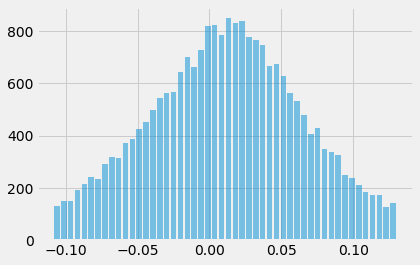In :
y.describe(percentiles=np.arange(.1, .91, .1).round(1))

Out:
count    22918.000000
mean         0.011216
std          0.052180
min         -0.108526
10%         -0.060198
20%         -0.035312
30%         -0.016502
40%         -0.001121
50%          0.012264
60%          0.025281
70%          0.039624
80%          0.056687
90%          0.080529
max          0.130222
Name: returns, dtype: float64
In :
total = len(y_binary)
plt.figure(figsize=(7,5))
g = sns.countplot(x=y_binary.values)
g.set_ylabel('Count', fontsize=14)
for p in g.patches:
height = p.get_height()
g.text(p.get_x()+p.get_width()/2.,
height + 1.5,
'{:1.2f}%'.format(height/total*100),
ha="center", fontsize=14, fontweight='bold')
plt.margins(y=0.1)
plt.title('Y Binary')
plt.show()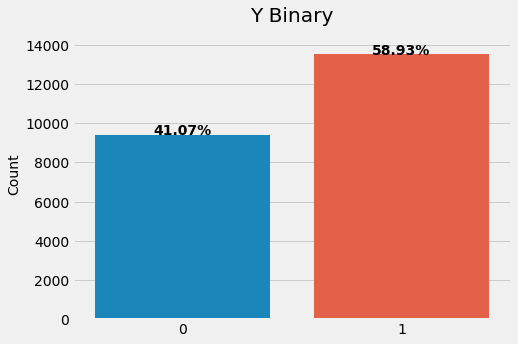## Custom KFold¶

Custom cross-validation class tailored to the format of the data just created, which has pandas MultiIndex with two levels, one for the ticker and one for the data.

OneStepTimeSeriesSplit ensures a split of training and validation sets that avoids a lookahead bias by training models using only data up to period $T-1$ for each stock when validating using data for month $T$. We will only use one-step-ahead forecasts.

In :
class OneStepTimeSeriesSplit():

def __init__(self, n_splits=3, test_period_length=1, shuffle=False):
self.n_splits = n_splits
self.test_period_length = test_period_length
self.shuffle = shuffle
self.test_end = n_splits * test_period_length

@staticmethod
def chunks(l, chunk_size):
for i in range(0, len(l), chunk_size):
yield l[i:i + chunk_size]

def split(self, X, y=None, groups=None):
unique_dates = (X.index
.get_level_values('date')
.unique()
.sort_values(ascending=False)[:self.test_end])

dates = X.reset_index()[['date']]
for test_date in self.chunks(unique_dates, self.test_period_length):
train_idx = dates[dates.date < min(test_date)].index
test_idx = dates[dates.date.isin(test_date)].index
if self.shuffle:
np.random.shuffle(list(train_idx))
yield train_idx, test_idx

def get_n_splits(self, X, y, groups=None):
return self.n_splits


### Functions to return an evaluation metric for a classifier or regressor using the custom cv class¶

In :
def regression_benchmark():
rmse = []
for train_idx, test_idx in cv.split(X):
mean = y.iloc[train_idx].mean()
data = y.iloc[test_idx].to_frame('y_test').assign(y_pred=mean)
rmse.append(np.sqrt(mean_squared_error(data.y_test, data.y_pred)))
return np.mean(rmse)

In :
def classification_benchmark():
auc = []
for train_idx, test_idx in cv.split(X):
mean = y_binary.iloc[train_idx].mean()
data = y_binary.iloc[test_idx].to_frame('y_test').assign(y_pred=mean)
auc.append(roc_auc_score(data.y_test, data.y_pred))
return np.mean(auc)


## View CV Dates & Sizes¶

In :
tss = OneStepTimeSeriesSplit(n_splits=12)
count = 0
for tr_idx, val_idx in tss.split(X2, y):
count += 1
if count == 4:
break
X_tr, X_vl = X2.iloc[tr_idx], X2.iloc[val_idx]
print(f'{len(X_tr)} data points for training:')
print(X_tr.info())
print()
print(f'{len(X_vl)} data points for testing:')
print(X_vl.info())
print()

22709 data points for training:
<class 'pandas.core.frame.DataFrame'>
MultiIndex: 22709 entries, (ABT, 2011-02-28 00:00:00) to (ZBRA, 2019-12-31 00:00:00)
Data columns (total 2 columns):
t-1    22709 non-null float64
t-2    22709 non-null float64
dtypes: float64(2)
memory usage: 424.2+ KB
None

209 data points for testing:
<class 'pandas.core.frame.DataFrame'>
MultiIndex: 209 entries, (ABT, 2020-01-31 00:00:00) to (ZBRA, 2020-01-31 00:00:00)
Data columns (total 2 columns):
t-1    209 non-null float64
t-2    209 non-null float64
dtypes: float64(2)
memory usage: 6.7+ KB
None

22478 data points for training:
<class 'pandas.core.frame.DataFrame'>
MultiIndex: 22478 entries, (ABT, 2011-02-28 00:00:00) to (ZBRA, 2019-11-30 00:00:00)
Data columns (total 2 columns):
t-1    22478 non-null float64
t-2    22478 non-null float64
dtypes: float64(2)
memory usage: 419.9+ KB
None

231 data points for testing:
<class 'pandas.core.frame.DataFrame'>
MultiIndex: 231 entries, (ABT, 2019-12-31 00:00:00) to (ZBRA, 2019-12-31 00:00:00)
Data columns (total 2 columns):
t-1    231 non-null float64
t-2    231 non-null float64
dtypes: float64(2)
memory usage: 7.1+ KB
None

22248 data points for training:
<class 'pandas.core.frame.DataFrame'>
MultiIndex: 22248 entries, (ABT, 2011-02-28 00:00:00) to (ZBRA, 2019-09-30 00:00:00)
Data columns (total 2 columns):
t-1    22248 non-null float64
t-2    22248 non-null float64
dtypes: float64(2)
memory usage: 415.7+ KB
None

230 data points for testing:
<class 'pandas.core.frame.DataFrame'>
MultiIndex: 230 entries, (ABT, 2019-11-30 00:00:00) to (ZBRA, 2019-11-30 00:00:00)
Data columns (total 2 columns):
t-1    230 non-null float64
t-2    230 non-null float64
dtypes: float64(2)
memory usage: 7.1+ KB
None



## Simple Regression Tree¶

Regression trees make predictions based on the mean outcome value for the training samples assigned to a given node and typically rely on the mean-squared error (MSE) to select optimal rules during recursive binary splitting.

Given a training set, the algorithm iterates over the predictors, $X_1, X_2, ..., X_p$, and possible cutpoints, $s_1, s_2, ..., s_N$, to find an optimal combination. The optimal rule splits the feature space into two regions, $\{X\mid X_i < s_j\}$ and $\{X\mid X_i > s_j\}$, with values for the $X_i$ feature either below or above the $s_j$ threshold so that predictions based on the training subsets maximize the reduction of the squared residuals relative to the current node.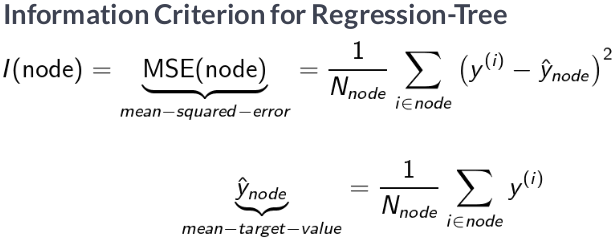### Configure Tree¶

We start with a simplified example to facilitate visualization and only use $2$ months of lagged returns to predict the following month, similar to an $AR(2)$ model.

In :
reg_tree_t2 = DecisionTreeRegressor(criterion='mse',
splitter='best',
max_depth=4,
min_samples_split=2,
min_samples_leaf=1,
min_weight_fraction_leaf=0.0,
max_features=None,
random_state=804,
max_leaf_nodes=None,
min_impurity_decrease=0.0,
min_impurity_split=None,
presort=False)


### Train/fit Decision Tree¶

In :
reg_tree_t2.fit(X=X2, y=y)

Out:
DecisionTreeRegressor(ccp_alpha=0.0, criterion='mse', max_depth=4,
max_features=None, max_leaf_nodes=None,
min_impurity_decrease=0.0, min_impurity_split=None,
min_samples_leaf=1, min_samples_split=2,
min_weight_fraction_leaf=0.0, presort=False,
random_state=804, splitter='best')

### Visualize Tree¶

• We visualize the tree using the graphviz library because sklearn can output a description of the tree using the .dot language used by that library.
• You can configure the output to include feature and class labels and limit the number of levels to keep the chart readable, as follows:
In :
dot_data = export_graphviz(reg_tree_t2,
out_file=None,
feature_names=X2.columns,
max_depth=2,
filled=True,
rounded=True,
special_characters=True)
# Draw graph
graph = pydotplus.graph_from_dot_data(dot_data)

# Show graph
Image(graph.create_png())

Out: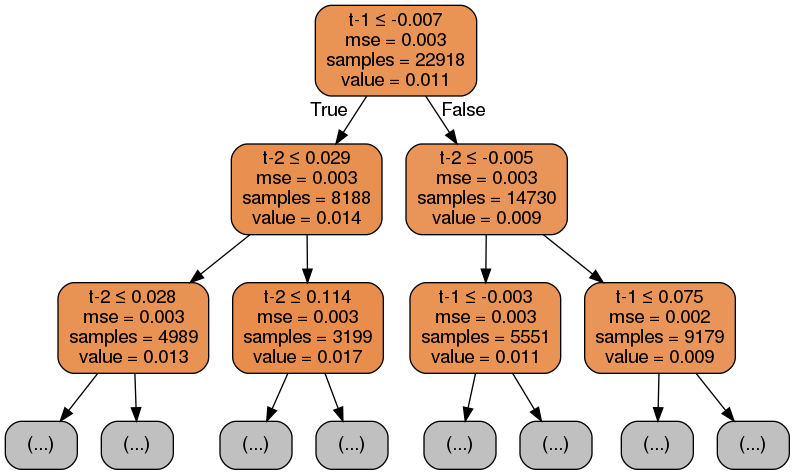### Save image¶

In :
# Create PDF
# graph.write_pdf("iris.pdf")

# Create PNG
graph.write_png("reg_tree_t2.png")

Out:
True

### Compare with Linear Regression¶

The OLS summary below and a visualization of the first two levels of the decision tree above reveal the striking differences between the models. The OLS model provides three parameters the slope and intercepts for the two features in line with the linear assumption.

In contrast, the regression tree chart above displays for each node of the first two levels the feature and threshold used to split the data (note that features can be used repeatedly), as well as the current value of the mean-squared error (MSE), the number of samples, and predicted value based on these training samples.

The tree chart also highlights the uneven distribution of samples across the nodes as the numbers vary between $14,700$ and $3,200$ samples after only two splits.

#### statsmodels OLS¶

In :
ols_model = sm.OLS(endog=y, exog=sm.add_constant(X2)).fit()
print(ols_model.summary())

                            OLS Regression Results
==============================================================================
Dep. Variable:                returns   R-squared:                       0.002
Method:                 Least Squares   F-statistic:                     23.45
Date:                Tue, 09 Jun 2020   Prob (F-statistic):           6.68e-11
Time:                        04:58:10   Log-Likelihood:                 35183.
No. Observations:               22918   AIC:                        -7.036e+04
Df Residuals:                   22915   BIC:                        -7.034e+04
Df Model:                           2
Covariance Type:            nonrobust
==============================================================================
coef    std err          t      P>|t|      [0.025      0.975]
------------------------------------------------------------------------------
const          0.0118      0.000     32.534      0.000       0.011       0.012
t-1           -0.0453      0.007     -6.849      0.000      -0.058      -0.032
t-2           -0.0020      0.007     -0.308      0.758      -0.015       0.011
==============================================================================
Omnibus:                      533.169   Durbin-Watson:                   2.002
Prob(Omnibus):                  0.000   Jarque-Bera (JB):              280.149
Skew:                          -0.048   Prob(JB):                     1.47e-61
Kurtosis:                       2.467   Cond. No.                         19.6
==============================================================================

Warnings:
 Standard Errors assume that the covariance matrix of the errors is correctly specified.


### Compare with Linear Time Series Models¶

#### statsmodels AR(2) Model¶

The coefficients are slightly different because an AR model treats returns as a single time series instead creating groups by ticker.

In :
ar_model = sm.tsa.ARMA(endog=y, order=(2,0)).fit()

In :
pd.DataFrame({'AR(2)': ar_model.params.values,
'OLS': ols_model.params.values},
index=ols_model.params.index)

Out:
AR(2) OLS
const 0.011216 0.011756
t-1 -0.045600 -0.045324
t-2 -0.004023 -0.002036
In :
ar_preds = ar_model.predict()


#### ARMA(2,2)¶

In :
arma_model = sm.tsa.ARMA(endog=y, order=(2, 2)).fit()
print(arma_model.summary())

                              ARMA Model Results
==============================================================================
Dep. Variable:                returns   No. Observations:                22918
Model:                     ARMA(2, 2)   Log Likelihood               35184.056
Method:                       css-mle   S.D. of innovations              0.052
Date:                Tue, 09 Jun 2020   AIC                         -70356.112
Time:                        04:58:59   BIC                         -70307.873
Sample:                             0   HQIC                        -70340.433

=================================================================================
coef    std err          z      P>|z|      [0.025      0.975]
---------------------------------------------------------------------------------
const             0.0112      0.000     34.871      0.000       0.011       0.012
ar.L1.returns     0.5877      0.731      0.804      0.422      -0.845       2.021
ar.L2.returns     0.0863      0.161      0.536      0.592      -0.229       0.402
ma.L1.returns    -0.6334      0.731     -0.866      0.386      -2.066       0.799
ma.L2.returns    -0.0621      0.184     -0.338      0.735      -0.422       0.298
Roots
=============================================================================
Real          Imaginary           Modulus         Frequency
-----------------------------------------------------------------------------
AR.1            1.4096           +0.0000j            1.4096            0.0000
AR.2           -8.2177           +0.0000j            8.2177            0.5000
MA.1            1.3895           +0.0000j            1.3895            0.0000
MA.2          -11.5923           +0.0000j           11.5923            0.5000
-----------------------------------------------------------------------------

In :
arma_preds = arma_model.predict()

In :
preds = X2.assign(arma=arma_preds, ar=ar_preds).sample(frac=.1).sort_values(['t-1', 't-2'])
preds.info()

<class 'pandas.core.frame.DataFrame'>
MultiIndex: 2292 entries, (DISCA, 2018-07-31 00:00:00) to (CI, 2015-08-31 00:00:00)
Data columns (total 4 columns):
t-1     2292 non-null float64
t-2     2292 non-null float64
arma    2292 non-null float64
ar      2292 non-null float64
dtypes: float64(4)
memory usage: 81.2+ KB

In :
q = 20
preds['t-1q'] = pd.qcut(preds['t-1'], q=q, labels=list(range(1, q+1))).astype(int)
preds['t-2q'] = pd.qcut(preds['t-2'], q=q, labels=list(range(1, q+1))).astype(int)


#### Decision Surfaces¶

The plot of the decision surface for both time series models illustrates how the ARMA model is capable of representing a more complex dynamic relationship.

In :
fig, axes = plt.subplots(ncols=2, figsize=(13, 6))

sns.heatmap(preds.groupby(['t-1q', 't-2q']).ar.median().unstack(), ax=axes, cmap='BuPu_r')
axes.set_title('AR(2) Model')
sns.heatmap(preds.groupby(['t-1q', 't-2q']).arma.median().unstack(), ax=axes, cmap='BuPu_r')
axes.set_title('ARMA(2,2) Model')

b, t = axes.set_ylim()
b += 0.5
t -= 0.5
axes.set_ylim(b, t)

b, t = axes.set_ylim()
b += 0.5
t -= 0.5
axes.set_ylim(b, t)

fig.tight_layout();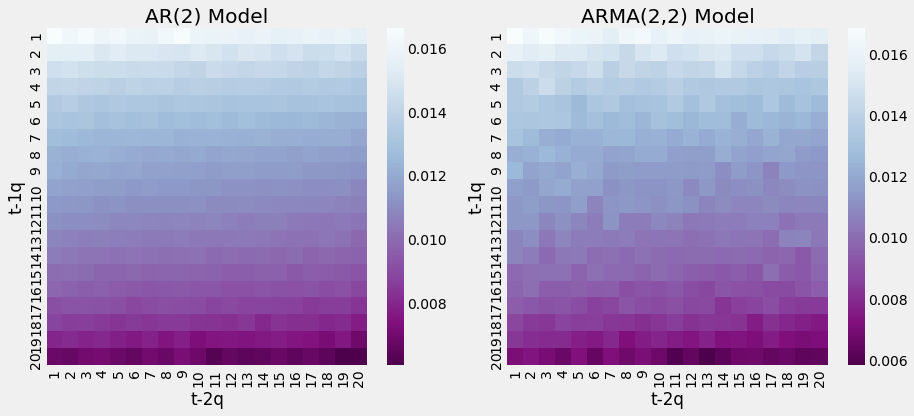### sklearn Linear Regression¶

In :
lin_reg = LinearRegression()

In :
lin_reg.fit(X=X2,y=y)

Out:
LinearRegression(copy_X=True, fit_intercept=True, n_jobs=None, normalize=False)
In :
lin_reg.intercept_

Out:
0.011755960724628655
In :
lin_reg.coef_

Out:
array([-0.04532443, -0.00203565])

### Linear Regression vs Regressin Tree Decision Surfaces¶

To further illustrate the different assumptions about the functional form of the relationships between the input variables and the output, we can visualize current return predictions as a function of the feature space, that is, as a function of the range of values for the lagged returns (features). The following figure shows the current period return as a function of returns one and two periods ago for linear regression and the regression tree:

The linear-regression model result on the left side underlines the linearity of the relationship between lagged and current returns, whereas the regression tree chart on the right illustrates the non-linear relationship encoded in the recursive partitioning of the feature space.

In :
t1, t2 = np.meshgrid(np.linspace(X2['t-1'].quantile(.01), X2['t-1'].quantile(.99), 100),
np.linspace(X2['t-2'].quantile(.01), X2['t-2'].quantile(.99), 100))

X_data = np.c_[t1.ravel(), t2.ravel()]

In :
fig, axes = plt.subplots(ncols=2, figsize=(13,5))

# Linear Regression
ret1 = lin_reg.predict(X_data).reshape(t1.shape)
surface1 = axes.contourf(t1, t2, ret1, cmap='coolwarm')
plt.colorbar(mappable=surface1, ax=axes)

# Regression Tree
ret2 = reg_tree_t2.predict(X_data).reshape(t1.shape)
surface2 = axes.contourf(t1, t2, ret2, cmap='coolwarm')
plt.colorbar(mappable=surface2, ax=axes)

# Format plots
titles = ['Linear Regression', 'Regression Tree']
for i, ax in enumerate(axes):
ax.set_xlabel('t-1')
ax.set_ylabel('t-2')
ax.set_title(titles[i])

fig.suptitle('Decision Surfaces', fontsize=20)
fig.tight_layout()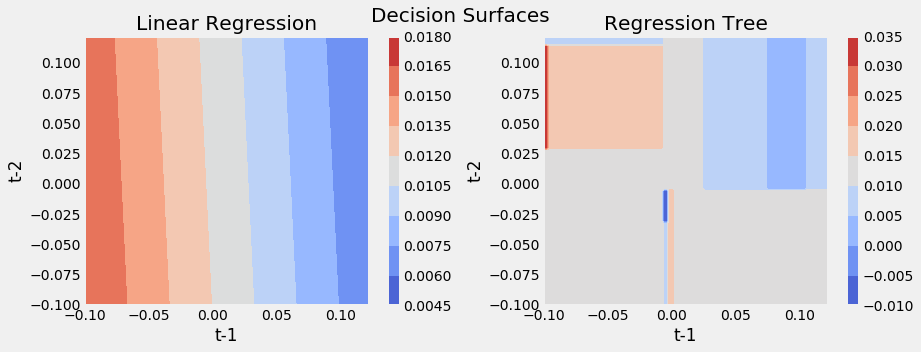## Basic Classification Tree¶

A classification tree works just like the regression version, except that categorical nature of the outcome requires a different approach to making predictions and measuring the loss. While a regression tree predicts the response for an observation assigned to a leaf node using the mean outcome of the associated training samples, a classification tree instead uses the mode, that is, the most common class among the training samples in the relevant region. A classification tree can also generate probabilistic predictions based on relative class frequencies.

### Loss Functions¶

When growing a classification tree, we also use recursive binary splitting but, instead of evaluating the quality of a decision rule using the reduction of the mean-squared error.

• Classification error rate: The fraction of the training samples in a given (leave) node that do not belong to the most common class.

Alternative measures:

Alternative measures are preferred because they are more sensitive to node purity than the classification error rate. Node purity refers to the extent of the quantity of a single class in a node. A node that only contains samples with outcomes belonging to a single class is pure and imply successful classification.

• Gini Impurity is a measurement of the likelihood of an incorrect classification of a new instance of a random variable, if that new instance were randomly classified according to the distribution of class labels from the training subset.

• Gini impurity is lower bounded by $0$, with $0$ occurring if the data set contains only one class.
• Information Gain, or IG for short, measures the reduction in entropy or surprise by splitting a dataset according to a given value of a random variable. A larger information gain suggests a lower entropy group or groups of samples, and hence less surprise.
• Entropy quantifies how much information there is in a random variable, or more specifically its probability distribution. A skewed distribution has a low entropy, a distribution where events have equal probability will have larger entropy.

Where classification outcomes take on $K$ values, $0,1,\dots,K-1$, for a given node, $m$, that represents a region, $R_m$, of the feature space and where $p_{mk}$ is the proportion of outcomes of the $k$ class in the $m$ node:

$$Entropy = -\sum_{k} P_{mk} log(p_{mk})$$$$Gini = \sum_{k} P_{mk} (1 - p_{mk})$$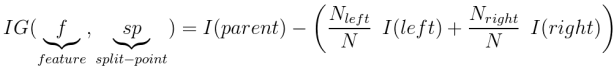In :
def entropy(f):
return (-f*np.log2(f) - (1-f)*np.log2(1-f))/2

In :
def gini(f):
return 2*f*(1-f)

In :
def misclassification_rate(f):
return np.where(f<=.5, f, 1-f)


Both the Gini Impurity and the Cross-Entropy measure take on smaller values when the class proportions approach zero or one, that is, when the child nodes become pure as a result of the split and are highest when the class proportions are even or $0.5$ in the binary case.

The chart below visualizes the values assumed by these two measures and the misclassification error rates across the $[0, 1]$ interval of proportions.

In :
x = np.linspace(0, 1, 10000)
(pd.DataFrame({'Gini': gini(x),
'Entropy': entropy(x),
'Misclassification Rate': misclassification_rate(x)}, index=x)
.plot(title='Classification Loss Functions', lw=2, figsize=(10,6)));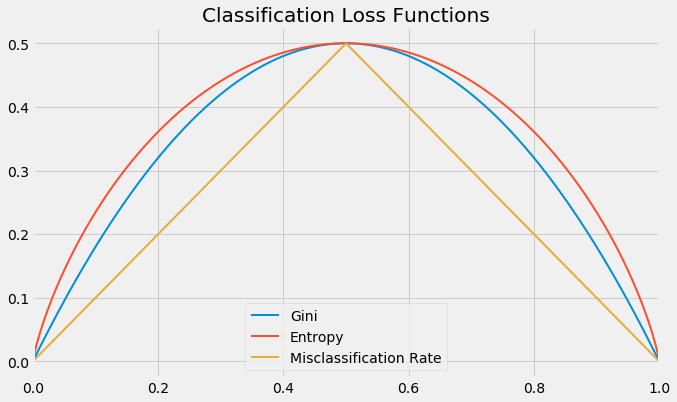#### Compare computation time¶

Gini is often preferred over entropy because it computes faster:

In :
%%timeit
misclassification_rate(x)

16.4 µs ± 458 ns per loop (mean ± std. dev. of 7 runs, 100000 loops each)

In :
%%timeit
gini(x)

12.3 µs ± 232 ns per loop (mean ± std. dev. of 7 runs, 100000 loops each)

In :
%%timeit
entropy(x)

220 µs ± 6.85 µs per loop (mean ± std. dev. of 7 runs, 1000 loops each)


### Configure Tree¶

In :
clf_tree_t2 = DecisionTreeClassifier(criterion='gini',
splitter='best',
max_depth=4,
min_samples_split=2,
min_samples_leaf=1,
min_weight_fraction_leaf=0.0,
max_features=None,
random_state=804,
max_leaf_nodes=None,
min_impurity_decrease=0.0,
min_impurity_split=None,
class_weight=None,
presort=False)


### Train Tree¶

In :
clf_tree_t2.fit(X=X2, y=y_binary)

Out:
DecisionTreeClassifier(ccp_alpha=0.0, class_weight=None, criterion='gini',
max_depth=4, max_features=None, max_leaf_nodes=None,
min_impurity_decrease=0.0, min_impurity_split=None,
min_samples_leaf=1, min_samples_split=2,
min_weight_fraction_leaf=0.0, presort=False,
random_state=804, splitter='best')

### Visualize Tree¶

In :
dot_data = export_graphviz(clf_tree_t2,
out_file=None,
feature_names=X2.columns,
class_names=['Down', 'Up'],
max_depth=2,
filled=True,
rounded=True,
special_characters=True)

# Draw graph
graph = pydotplus.graph_from_dot_data(dot_data)

# Show graph
Image(graph.create_png())

Out: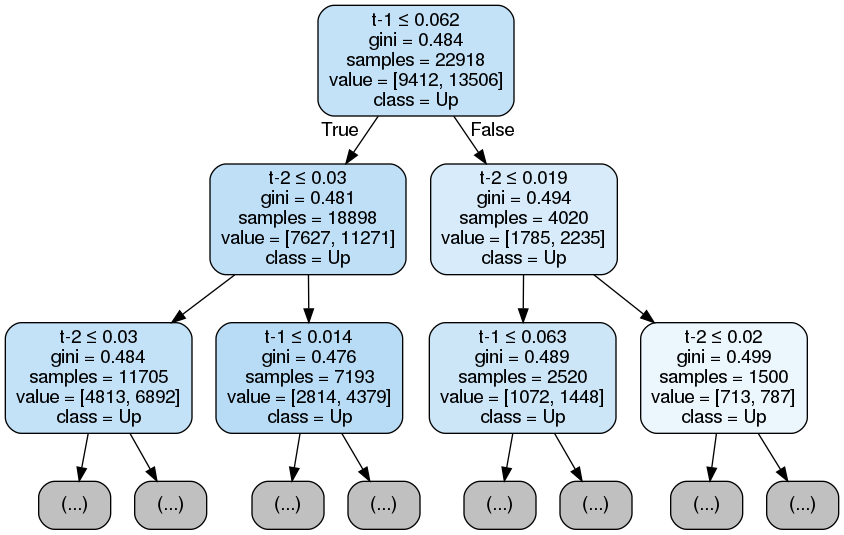### Compare with Logistic Regression¶

#### Statsmodels¶

In :
model = sm.Logit(endog=y_binary, exog=sm.add_constant(X2)).fit()
print(model.summary())

Optimization terminated successfully.
Current function value: 0.676736
Iterations 4
Logit Regression Results
==============================================================================
Dep. Variable:                returns   No. Observations:                22918
Model:                          Logit   Df Residuals:                    22915
Method:                           MLE   Df Model:                            2
Date:                Tue, 09 Jun 2020   Pseudo R-squ.:               0.0005455
Time:                        05:00:34   Log-Likelihood:                -15509.
converged:                       True   LL-Null:                       -15518.
Covariance Type:            nonrobust   LLR p-value:                 0.0002107
==============================================================================
coef    std err          z      P>|z|      [0.025      0.975]
------------------------------------------------------------------------------
const          0.3720      0.014     26.342      0.000       0.344       0.400
t-1           -1.0489      0.258     -4.060      0.000      -1.555      -0.543
t-2            0.1215      0.258      0.471      0.637      -0.384       0.626
==============================================================================


#### sklearn¶

In :
logistic_reg = LogisticRegression()

In :
logistic_reg.fit(X=X2, y=y_binary)

Out:
LogisticRegression(C=1.0, class_weight=None, dual=False, fit_intercept=True,
intercept_scaling=1, l1_ratio=None, max_iter=100,
multi_class='auto', n_jobs=None, penalty='l2',
random_state=None, solver='lbfgs', tol=0.0001, verbose=0,
warm_start=False)
In :
logistic_reg.coef_

Out:
array([[-0.98361778,  0.11664984]])

### Plot Decision Surfaces¶

In :
fig, axes = plt.subplots(ncols=2, figsize=(12,5))

# Linear Regression
ret1 = logistic_reg.predict_proba(X_data)[:, 1].reshape(t1.shape)
surface1 = axes.contourf(t1, t2, ret1, cmap='coolwarm')
plt.colorbar(mappable=surface1, ax=axes)

# Regression Tree
ret2 = clf_tree_t2.predict_proba(X_data)[:, 1].reshape(t1.shape)
surface2 = axes.contourf(t1, t2, ret2, cmap='coolwarm')
plt.colorbar(mappable=surface2, ax=axes)

# Format plots
titles = ['Logistic Regression', 'Classification Tree']
for i, ax in enumerate(axes):
ax.set_xlabel('t-1')
ax.set_ylabel('t-2')
ax.set_title(titles[i])

fig.suptitle('Decision Surfaces', fontsize=20)
fig.tight_layout()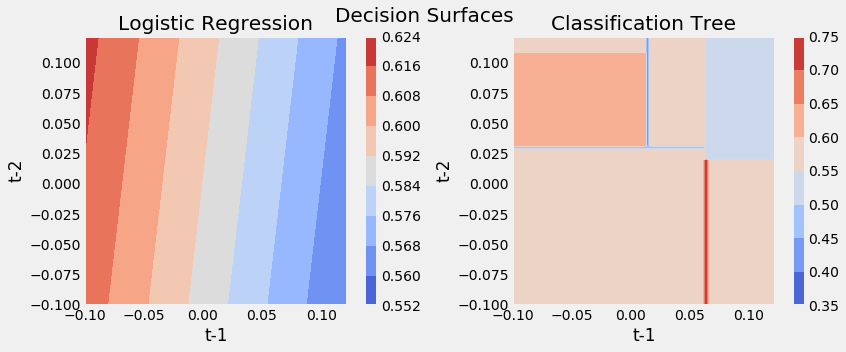## Regression Tree with all Features¶

We now train, visualize, and evaluate a regression tree with up to $5$ consecutive splits using $80\%$ of the samples for training to predict the remaining $20\%$.

We are taking a shortcut here to simplify the illustration and use the built-in train_test_split, which does not protect against lookahead bias, as our custom cv class iterator. The tree configuration implies up to $2^5=32$ leaf nodes that, on average in the balanced case, would contain over $4,300$ of the training samples.

### Train-Test Split¶

In :
X_train, X_test, y_train, y_test = train_test_split(X, y, test_size=0.2, random_state=804)


### Configure Tree¶

The output after training the model displays all the DecisionTreeClassifier parameters that we will address in more detail in the next section when we discuss parameter-tuning.

In :
regression_tree = DecisionTreeRegressor(criterion='mse',
splitter='best',
max_depth=5,
min_samples_split=2,
min_samples_leaf=1,
min_weight_fraction_leaf=0.0,
max_features=None,
random_state=804,
max_leaf_nodes=None,
min_impurity_decrease=0.0,
min_impurity_split=None,
presort=False)


### Train Model¶

In :
regression_tree.fit(X=X_train, y=y_train)

Out:
DecisionTreeRegressor(ccp_alpha=0.0, criterion='mse', max_depth=5,
max_features=None, max_leaf_nodes=None,
min_impurity_decrease=0.0, min_impurity_split=None,
min_samples_leaf=1, min_samples_split=2,
min_weight_fraction_leaf=0.0, presort=False,
random_state=804, splitter='best')

### Visualize Tree¶

The result shows that the model uses a variety of different features and indicates the split rules for both continuous and categorical (dummy) variables.

In :
dot_data = export_graphviz(regression_tree,
out_file=None,
feature_names=X_train.columns,
max_depth=3,
filled=True,
rounded=True,
special_characters=True)
# Draw graph
graph = pydotplus.graph_from_dot_data(dot_data)

# Show graph
Image(graph.create_png())

Out: